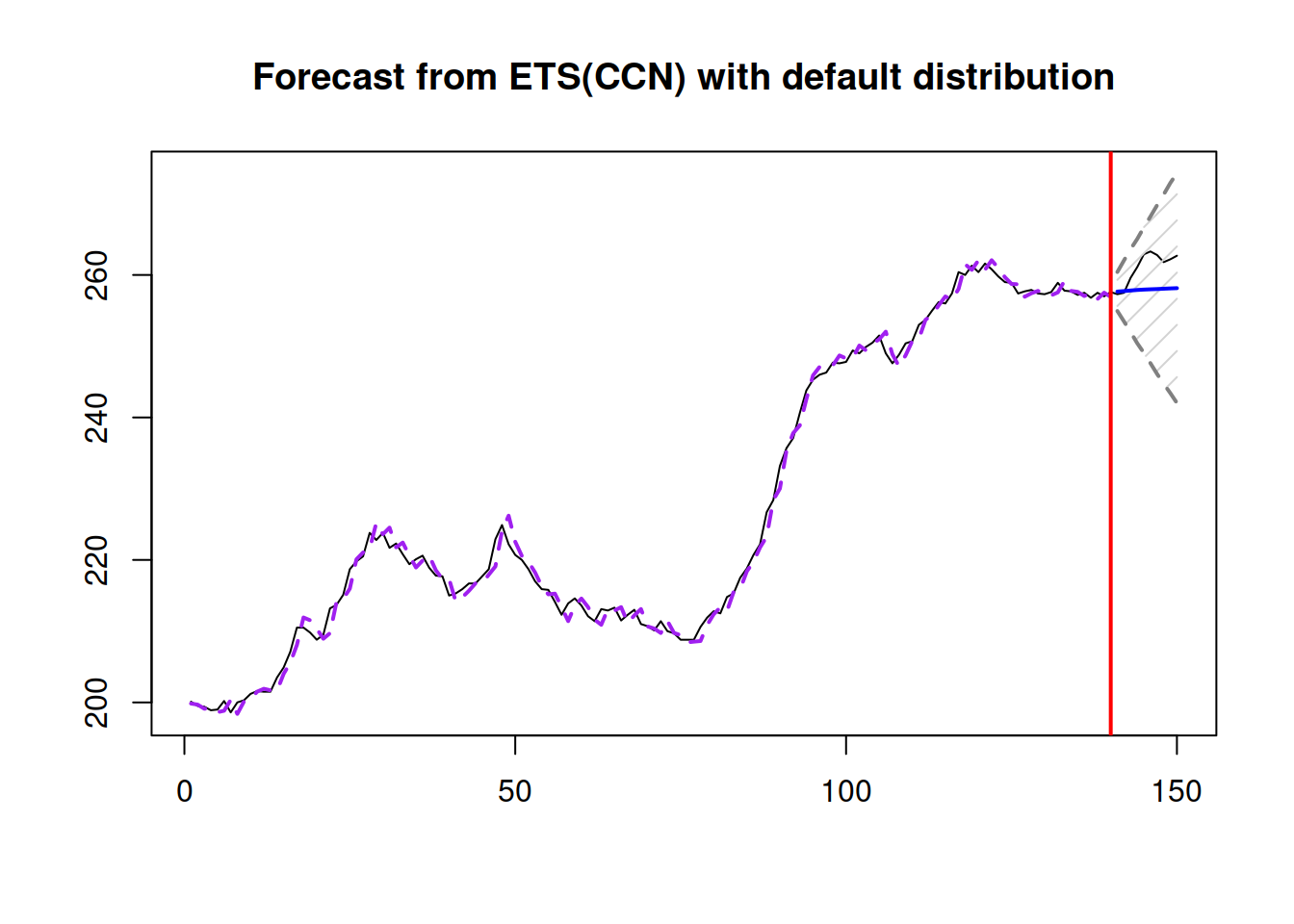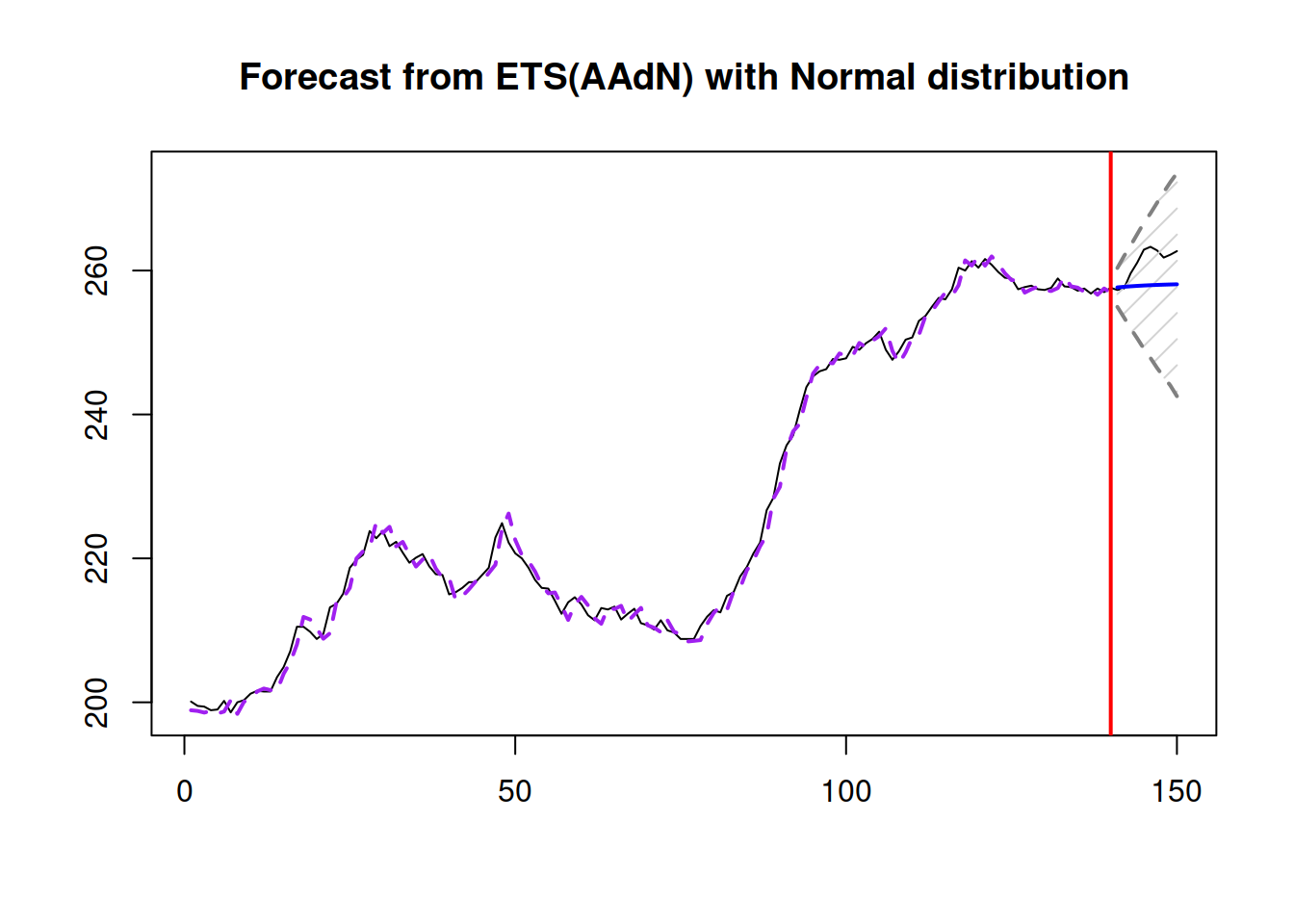This book is in Open Review. I want your feedback to make the book better for you and other readers. To add your annotation, select some text and then click the on the pop-up menu. To see the annotations of others, click the button in the upper right hand corner of the page

## 15.2 ADAM ARIMA order selection

While ETS has 30 models to choose from, ARIMA has many more options. For example, selecting the non-seasonal ARIMA with / without constant restricting the orders with $$p \leq 3$$, $$d \leq 2$$ and $$q \leq 3$$ leads to the combination of $$3 \times 2 \times 3 \times 2 = 36$$ possible ARIMA models. If we increase the possible orders to 5 or even more, we will need to go through hundreds of models. Adding the seasonal part increases this number by an order of magnitude. This means that we cannot just test all possible ARIMA models and select the most appropriate one, we need to be smart in the selection proces.

developed an efficient mechanism of ARIMA order selection based on statistical tests (for stationarity and seasonality), reducing the number of models to test to reasonable ammount. developed an alternative mechanism, relying purely on information criteria, which works especially well on seasonal data, but potentially may lead to models that overfit the data (this is implemented in auto.ssarima() and auto.msarima() functions in smooth package). We also have the Box-Jenkins approach for ARIMA orders selection, which relies on the analysis of ACF and PACF, but we should not forget the limitations of that approach. Finally, proposed the stepwise trace forward approach, which relies on partial correlations and uses the information criteria to test model on each iteration. Building upon all of that, I have developed the following algorithm for order selection of ADAM ARIMA:

1. Determine the order of differences by fitting all possible combinations of ARIMA models with $$P_j=0$$ and $$Q_j=0$$ for all lags $$j$$. This includes trying the models with and without the constant term. The order $$D_j$$ is then determined via the model with the lowest IC;
2. Then iteratively, starting from the highest seasonal lag and moving to the lag of 1 do for every lag $$m_j$$:
1. Calculate ACF of residuals of the model;
2. Find the highest value of autocorrelation coefficient that corresponds to the multiple of the respective seasonal lag $$m_j$$;
3. Define, what should be the order of MA based on the lag of the autocorrelation coefficient on the previous step and include it in the ARIMA model;
4. Calculate IC, and if it is lower than for the previous best model, keep the new MA order;
5. Repeat (a) - (d) while there is an improvement in IC;
6. Do steps (a) - (e) for AR order, substituting ACF with PACF of the residuals of the best model;
7. Move to the next seasonal lag;
1. Try out several restricted ARIMA models of the order $$q=d$$ (this is based on (1) and the restrictions provided by user). The motivation for this comes from the idea of relation between ARIMA and ETS.

As you can see, this algorithm relies on the idea of Box-Jenkins methodology, but takes it with a pinch of salt, checking every time if the proposed order is improving the model or not. The motivation for doing MA orders before AR is based on the understanding of what AR model implies for forecasting. In a way, it is safer to have ARIMA(0,d,q) model than ARIMA(p,d,0), because the former is less prone to overfitting than the latter. Finally, the proposed algorithm is faster than the algorithm of and is more modest in the number of selected orders of the model.

In order to start the algorithm, you would need to provide a parameter select=TRUE in the orders. Here is an example with Box-Jenkins data:

adamARIMAModel <- adam(BJsales, model="NNN",
orders=list(ar=3,i=2,ma=3,select=TRUE),
h=10, holdout=TRUE)

In this example, orders=list(ar=3,i=2,ma=3,select=TRUE) tells function that the maximum orders to check are $$p\leq 3$$, $$d\leq 2$$ $$q\leq 3$$. The resulting model is ARIMA(0,2,2) and has the following fit:

plot(adamARIMAModel,7)adamARIMAModel
## Time elapsed: 0.66 seconds
## Model estimated using auto.adam() function: ARIMA(0,2,2)
## Distribution assumed in the model: Normal
## Loss function type: likelihood; Loss function value: 243.2819
## ARMA parameters of the model:
## MA:
## theta1 theta2
##   -0.7515   -0.0109
##
## Sample size: 140
## Number of estimated parameters: 5
## Number of degrees of freedom: 135
## Information criteria:
##      AIC     AICc      BIC     BICc
## 496.5638 497.0115 511.2720 512.3783
##
## Forecast errors:
## ME: 3.224; MAE: 3.339; RMSE: 3.794
## sCE: 14.156%; Asymmetry: 91.6%; sMAE: 1.466%; sMSE: 0.028%
## MASE: 2.825; RMSSE: 2.488; rMAE: 0.927; rRMSE: 0.923

Note that when optimal initials are used, the resulting model will be parsimonious. If we want to have a more flexible model, we can use a different initialisation, and in some cases the algorithm will select a model with higher orders of AR, I and MA.

### 15.2.1 ETS + ARIMA restrictions

Based on the relation between ARIMA and ETS, when selecting ARIMA orders, we do not need to test some of combinations of models. For example, if we already consider ETS(A,N,N), then we do not need to check ARIMA(0,1,1) model. The recommendations for what to skip in different circumstances have been discussed in Section 9.4. Still there are different ways how to construct an ETS + ARIMA model, with different sequence between ETS selection / ARIMA selection. We suggest to start with ETS, then go to the selection of ARIMA orders. This way, we are building upon the robust forecasting model and see if it can be improved further by introducing elements that are not there. Note that given the complexity of the task of estimating all parameters for ETS and ARIMA, it is advised to use backcasting (see Section 11.4.1) for the initialisation of the model if a model selection is required for ETS + ARIMA. Here is an example in R:

adamETSARIMAModel <-
orders=list(ar=c(3,3),i=c(2,1),ma=c(3,3),select=TRUE),
h=10, holdout=TRUE, initial="back")

adamETSARIMAModel
## Time elapsed: 1.28 seconds
## Model estimated using auto.adam() function: ETS(MMM)+SARIMA(3,0,0)(1,0,0)
## Distribution assumed in the model: Gamma
## Loss function type: likelihood; Loss function value: 468.391
## Persistence vector g:
##  alpha   beta  gamma
## 0.5109 0.0046 0.0000
##
## ARMA parameters of the model:
## AR:
##  phi1  phi2  phi3 phi1
##   0.2154   0.2296  -0.0402   0.2084
##
## Sample size: 134
## Number of estimated parameters: 8
## Number of degrees of freedom: 126
## Information criteria:
##      AIC     AICc      BIC     BICc
## 952.7819 953.9339 975.9647 978.7858
##
## Forecast errors:
## ME: 3.416; MAE: 16.347; RMSE: 20.154
## sCE: 12.908%; Asymmetry: 31.9%; sMAE: 6.178%; sMSE: 0.58%
## MASE: 0.681; RMSSE: 0.646; rMAE: 0.164; rRMSE: 0.163
plot(adamETSARIMAModel,7)The resulting model is ETS(M,M,M) with AR elements: 3 non-seasonal ones and 1 seasonal AR, which improve the fit of the model and hopefully result in more accurate forecasts.

### References

• Hyndman, R.J., Khandakar, Y., 2008. Automatic time series forecasting: The forecast package for R. Journal of Statistical Software. 26, 1–22. https://www.jstatsoft.org/article/view/v027i03
• Sagaert, Y.R., Svetunkov, I., 2021. Variables Selection Using Partial Correlations and Information Criteria.
• Svetunkov, I., Boylan, J.E., 2020b. State-space ARIMA for supply-chain forecasting. International Journal of Production Research. 58, 818–827. https://doi.org/10.1080/00207543.2019.1600764